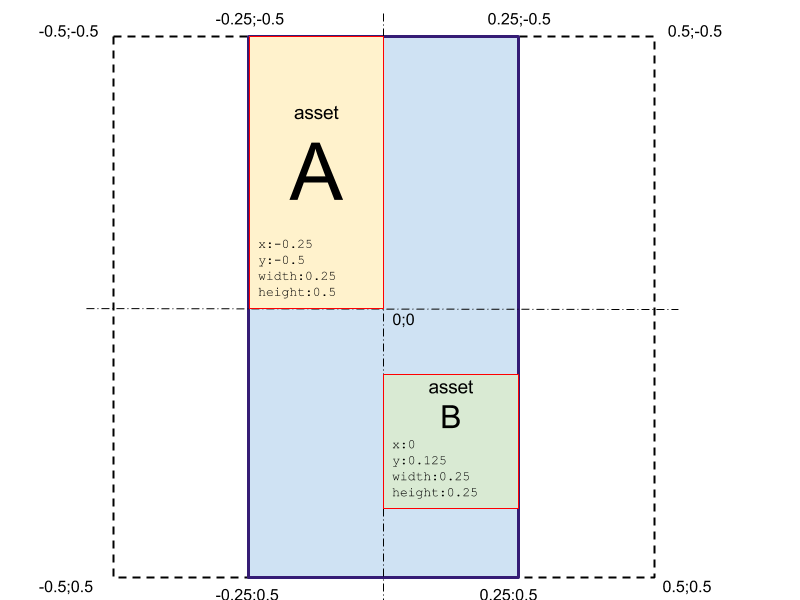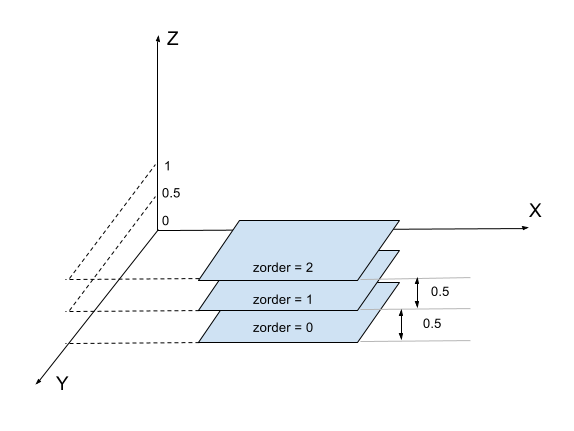# Assets sizing and positioning

For asset we have following attributes:

attribute description range
x left position of the markerﾕs top-left corner [-0.5:0.5]
y top position of the markerﾕs top-left corner [-0.5:0.5]
width assetﾕs width [0:1]
height assetﾕs height [0:1]

Assets size and position values are relative to marker's longest size.

That's if we have a marker width=200px and height=400px all the assets' attributes will be relative to marker's height (400px in our case).Here are some helpful formulas (W-markers width, H-marker's height, w-asset's width, h-asset's height):

top left corner of the marker x = -0.5 * min(W/H, 1) y = -0.5 * min(H/W, 1)
top right corner of the marker x = 0.5 * min(W/H, 1) y = 0.5 * min(H/W, 1)
bottom left corner of the marker x = -0.5 * min(W/H, 1) y = -0.5 * min(H/W, 1)
bottom right corner of the marker x = 0.5 * min(W/H, 1) y = 0.5 * min(H/W, 1)

### Mobile side positioning

#### Automatic z coordinate calculation

We have a problem for 2D assets with rendering assets with the same z coordinate (assets blinking). To avoid this we've decided to calculate z coordinate automatically by adding 0.5 to next asset in a set (ordered by z order).

This works for all 2D assets without is_3d_context and is_vertical flags.#### Vertical 2D assets (any NON 3D asset with is_vertical==1)

For those assets z coordinate calculated front end side using following formula: `z = 320 * (absolute(sinus(alpha)) * [asset height in px]/2 + [altitude in px]) / [marker width in px]`

where altitude is a distance (in pixels) between marker and bottom of the asset

Back altitude calculation from the z coordinate:

`altitude = z*[marker width in px] / 320- absolute(sinus(alpha)) * [asset height in px]/2`

#### Mobile side rendering

1. For 3d context asset - assets rendering by z_order (from low to high)
2. For plane assets - assets rendering by z coord, from high to low
3. For vertical assets - nothing changes, assets rendering by z coord, from high to low
Cet article vous a-t-il été utile ?
Utilisateurs qui ont trouvé cela utile : 0 sur 0

### Commentaires

0 commentaire

Vous devez vous connecter pour laisser un commentaire.